﻿ Meet this year's judges and finalistsLike   Tweet
 /* styles */ Dear Readers, This is an exciting time of year for the Dayton Literary Peace Prize: the announcement of the finalists in fiction and nonfiction for 2017 and the recognition of our final judges. Each year, our first readers winnow over one hundred nominations down to the six books in each category that best fit our guidelines (see Nomination Guidelines on our website). Our final judges, award-winning writers with international reputations, working in Oxford, England; Singapore; and here in the U.S., are now considering the finalists to determine the winner and runner-up in each category. Their decisions will be announced in early October. The powerful finalists will lead the reader to a better understanding of our world. Consider adding them to your fall reading lists.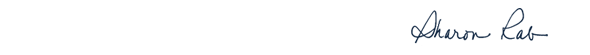table div table+table+table+table div table{width:100%;padding:0}table div table+table+table+table div table img{width:96.23%;padding:0;float:none}table div table+table+table+table div table td{width:100%;padding:0 1.88% 18px}/* styles */# Meet our Judges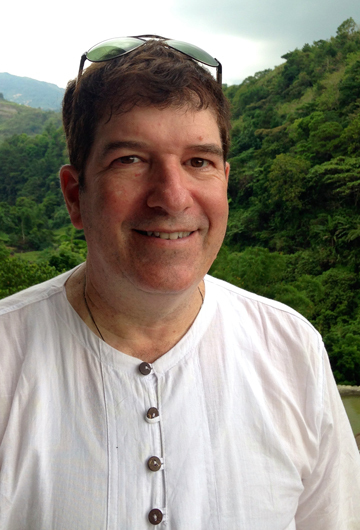Robin Hemley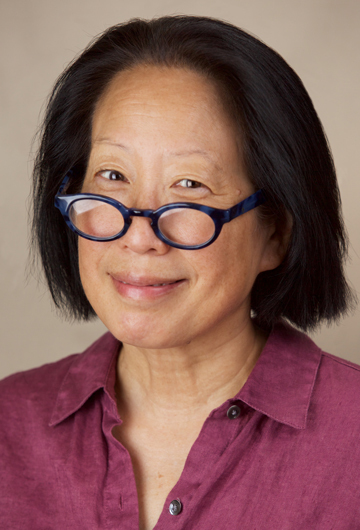Gish Jen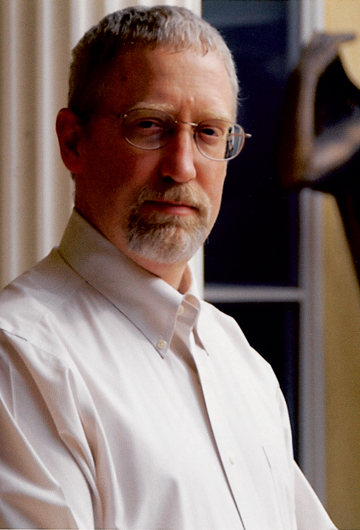Alan Taylor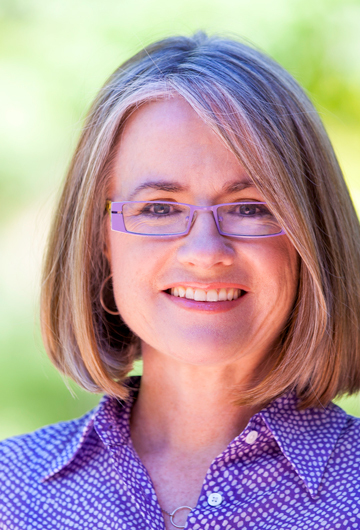Helen Thorp
 table div table+table+table+table+table+table+table div table{width:100%;padding:0}table div table+table+table+table+table+table+table div table img{width:96.23%;padding:0;float:none}table div table+table+table+table+table+table+table div table td{width:100%;padding:0 1.88% 18px}/* styles *//* styles */ Robin Hemley is the author of twelve books of nonfiction and fiction, and has won numerous awards for his writing, including a Guggenheim Fellowship, three Pushcart Prizes in both fiction and nonfiction, The Independent Press Book Award, an Editors Choice Award from The American Library Association, State Arts Council grants from Washington, North Carolina, and Illinois, The Ohioana Library Association Award, and fellowships from the Fine Arts Work Center in Provincetown, The MacDowell Colony, and Read more...
 table div table+table+table+table+table+table+table+table+table div table{width:100%;padding:0}table div table+table+table+table+table+table+table+table+table div table img{width:96.23%;padding:0;float:none}table div table+table+table+table+table+table+table+table+table div table td{width:100%;padding:0 1.88% 18px}/* styles *//* styles */ The author of six previous books, Gish Jen has published short work in The New Yorker, The Atlantic Monthly, and dozens of other periodicals and anthologies. Her work has appeared in The Best American Short Stories four times, including The Best American Short Stories of the Century. Nominated for a National Book Critics’ Circle Award, her work was featured in a PBS American Masters’ special on the American novel, and is widely taught. Read more...
 table div table+table+table+table+table+table+table+table+table+table+table div table{width:100%;padding:0}table div table+table+table+table+table+table+table+table+table+table+table div table img{width:96.23%;padding:0;float:none}table div table+table+table+table+table+table+table+table+table+table+table div table td{width:100%;padding:0 1.88% 18px}/* styles *//* styles */ Alan Taylor is an award-winning author and teacher. He taught in the history department at Boston University from 1987 to 1994. Since 1994, he has been a professor at the University of California at Davis. In 2002 he won the University of California at Davis Award for Teaching and Scholarly Achievement and the Phi Beta Kappa, Northern California Association, Teaching Excellence Award. Read more...
 table div table+table+table+table+table+table+table+table+table+table+table+table+table div table{width:100%;padding:0}table div table+table+table+table+table+table+table+table+table+table+table+table+table div table img{width:96.23%;padding:0;float:none}table div table+table+table+table+table+table+table+table+table+table+table+table+table div table td{width:100%;padding:0 1.88% 18px}/* styles *//* styles */ Helen Thorpe is an award-winning journalist who lives in Denver, Colorado. Her first book, Just Like Us: The True Story Of Four Mexican Girls Coming Of Age In America, was published in 2009. It won the Colorado Book Award and was named one of the best books of the year by the Washington Post. Read more...
 table div table+table+table+table+table+table+table+table+table+table+table+table+table+table+table div table{width:100%;padding:0}table div table+table+table+table+table+table+table+table+table+table+table+table+table+table+table div table img{width:96.23%;padding:0;float:none}table div table+table+table+table+table+table+table+table+table+table+table+table+table+table+table div table td{width:100%;padding:0 1.88% 18px}/* styles */# Our Finalists

 table div table+table+table+table+table+table+table+table+table+table+table+table+table+table+table+table+table div table{width:100%;padding:0}table div table+table+table+table+table+table+table+table+table+table+table+table+table+table+table+table+table div table img{width:96.23%;padding:0;float:none}table div table+table+table+table+table+table+table+table+table+table+table+table+table+table+table+table+table div table td{width:100%;padding:0 1.88% 18px}/* styles */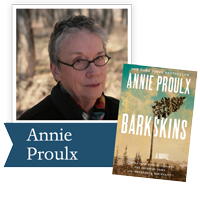Pulitzer Prize and National Book Award-winning author Annie Proulx’s most ambitious and epic work ever, a dazzling feat of imagination and research ten years in the writing—a violent, bloody, magnificently dramatic novel about the forming of the new world over 200 years ago.

 table div table+table+table+table+table+table+table+table+table+table+table+table+table+table+table+table+table+table+table div table{width:100%;padding:0}table div table+table+table+table+table+table+table+table+table+table+table+table+table+table+table+table+table+table+table div table img{width:96.23%;padding:0;float:none}table div table+table+table+table+table+table+table+table+table+table+table+table+table+table+table+table+table+table+table div table td{width:100%;padding:0 1.88% 18px}/* styles */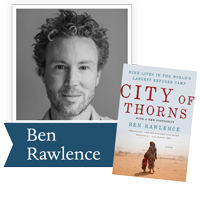In City of Thorns, Rawlence interweaves the stories of nine individuals to show what life is like in Dadaab, the world's largest refugee camp, sketching the wider political forces that keep the refugees trapped. Lucid, vivid, and illuminating, City of Thorns is an urgent human story with deep international repercussions, brought to life through the people who call Dabaab home.

 table div table+table+table+table+table+table+table+table+table+table+table+table+table+table+table+table+table+table+table+table+table div table{width:100%;padding:0}table div table+table+table+table+table+table+table+table+table+table+table+table+table+table+table+table+table+table+table+table+table div table img{width:96.23%;padding:0;float:none}table div table+table+table+table+table+table+table+table+table+table+table+table+table+table+table+table+table+table+table+table+table div table td{width:100%;padding:0 1.88% 18px}/* styles */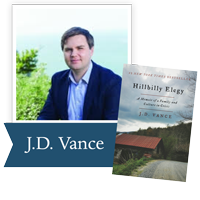From a former marine and Yale Law School graduate, a powerful account of growing up in a poor Rust Belt town that offers a broader, probing look at the struggles of America’s white working class.

 table div table+table+table+table+table+table+table+table+table+table+table+table+table+table+table+table+table+table+table+table+table+table+table div table{width:100%;padding:0}table div table+table+table+table+table+table+table+table+table+table+table+table+table+table+table+table+table+table+table+table+table+table+table div table img{width:96.23%;padding:0;float:none}table div table+table+table+table+table+table+table+table+table+table+table+table+table+table+table+table+table+table+table+table+table+table+table div table td{width:100%;padding:0 1.88% 18px}/* styles */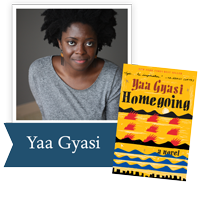A riveting, kaleidoscopic debut novel: a story of race, history, ancestry, love, and time that traces the descendants of two sisters torn apart in eighteenth-century Africa across three hundred years in Ghana and America.

 table div table+table+table+table+table+table+table+table+table+table+table+table+table+table+table+table+table+table+table+table+table+table+table+table+table div table{width:100%;padding:0}table div table+table+table+table+table+table+table+table+table+table+table+table+table+table+table+table+table+table+table+table+table+table+table+table+table div table img{width:96.23%;padding:0;float:none}table div table+table+table+table+table+table+table+table+table+table+table+table+table+table+table+table+table+table+table+table+table+table+table+table+table div table td{width:100%;padding:0 1.88% 18px}/* styles */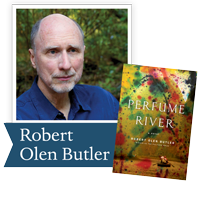In Perfume River, Pulitzer Prize-winning author Robert Olen Butler traces the legacy of the Vietnam War through the dramatic portrait of a single North Florida family struggling to confront the past. It is a profound and poignant book that echoes the American experience and the lives of so many affected by war.

 table div table+table+table+table+table+table+table+table+table+table+table+table+table+table+table+table+table+table+table+table+table+table+table+table+table+table+table div table{width:100%;padding:0}table div table+table+table+table+table+table+table+table+table+table+table+table+table+table+table+table+table+table+table+table+table+table+table+table+table+table+table div table img{width:96.23%;padding:0;float:none}table div table+table+table+table+table+table+table+table+table+table+table+table+table+table+table+table+table+table+table+table+table+table+table+table+table+table+table div table td{width:100%;padding:0 1.88% 18px}/* styles */Sly, funny, intelligent, and artfully structured, The Fortunes by Peter Ho Davies recasts American history through the lives of Chinese Americans and reimagines the multigenerational novel through the fractures of immigrant family experience.

 table div table+table+table+table+table+table+table+table+table+table+table+table+table+table+table+table+table+table+table+table+table+table+table+table+table+table+table+table+table div table{width:100%;padding:0}table div table+table+table+table+table+table+table+table+table+table+table+table+table+table+table+table+table+table+table+table+table+table+table+table+table+table+table+table+table div table img{width:96.23%;padding:0;float:none}table div table+table+table+table+table+table+table+table+table+table+table+table+table+table+table+table+table+table+table+table+table+table+table+table+table+table+table+table+table div table td{width:100%;padding:0 1.88% 18px}/* styles */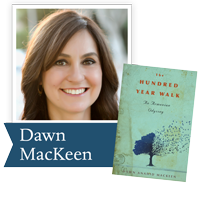In The Hundred-Year Walk, Dawn MacKeen alternates between her grandfather Stepan’s courageous account of surviving the Armenian genocide of 1915, drawn from his long-lost journals, and her own story as she attempts to retrace his steps, setting out alone to Turkey and Syria, shadowing her resourceful, resilient grandfather across a landscape still rife with tension. Their shared story is a testament to family, to home, and to the power of the human spirit to transcend the barriers of religion, ethnicity, and even time itself.

 table div table+table+table+table+table+table+table+table+table+table+table+table+table+table+table+table+table+table+table+table+table+table+table+table+table+table+table+table+table+table+table div table{width:100%;padding:0}table div table+table+table+table+table+table+table+table+table+table+table+table+table+table+table+table+table+table+table+table+table+table+table+table+table+table+table+table+table+table+table div table img{width:96.23%;padding:0;float:none}table div table+table+table+table+table+table+table+table+table+table+table+table+table+table+table+table+table+table+table+table+table+table+table+table+table+table+table+table+table+table+table div table td{width:100%;padding:0 1.88% 18px}/* styles */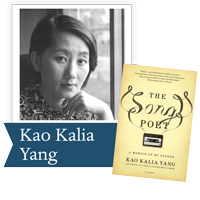Written with the exquisite beauty for which Kao Kalia Yang is renowned, The Song Poet recounts the life of her father Bee Yang, a Hmong refugee in Minnesota, driven from the mountains of Laos by American's Secret War. Above all, it is a love story—of a daughter for her father, a father for his children, a people for their land, their traditions, and all that they have lost.

 table div table+table+table+table+table+table+table+table+table+table+table+table+table+table+table+table+table+table+table+table+table+table+table+table+table+table+table+table+table+table+table+table+table div table{width:100%;padding:0}table div table+table+table+table+table+table+table+table+table+table+table+table+table+table+table+table+table+table+table+table+table+table+table+table+table+table+table+table+table+table+table+table+table div table img{width:96.23%;padding:0;float:none}table div table+table+table+table+table+table+table+table+table+table+table+table+table+table+table+table+table+table+table+table+table+table+table+table+table+table+table+table+table+table+table+table+table div table td{width:100%;padding:0 1.88% 18px}/* styles */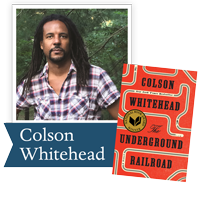Winner of the Pulitzer Prize and the National Book Award, the #1 New York Times bestseller from Colson Whitehead, a magnificent tour de force chronicling a young slave's adventures as she makes a desperate bid for freedom in the antebellum South.

 table div table+table+table+table+table+table+table+table+table+table+table+table+table+table+table+table+table+table+table+table+table+table+table+table+table+table+table+table+table+table+table+table+table+table+table div table{width:100%;padding:0}table div table+table+table+table+table+table+table+table+table+table+table+table+table+table+table+table+table+table+table+table+table+table+table+table+table+table+table+table+table+table+table+table+table+table+table div table img{width:96.23%;padding:0;float:none}table div table+table+table+table+table+table+table+table+table+table+table+table+table+table+table+table+table+table+table+table+table+table+table+table+table+table+table+table+table+table+table+table+table+table+table div table td{width:100%;padding:0 1.88% 18px}/* styles */From award-winning author, Patricia Engel, The Veins of the Ocean follows the riveting story of one young woman’s devotion to her brother on death row and the journey she takes toward a freer future. Set along the vibrant coasts of Miami, Havana, and Cartagena, this novel explores the beauty of the natural world and the solace it brings to even the most fractured lives.

 table div table+table+table+table+table+table+table+table+table+table+table+table+table+table+table+table+table+table+table+table+table+table+table+table+table+table+table+table+table+table+table+table+table+table+table+table+table div table{width:100%;padding:0}table div table+table+table+table+table+table+table+table+table+table+table+table+table+table+table+table+table+table+table+table+table+table+table+table+table+table+table+table+table+table+table+table+table+table+table+table+table div table img{width:96.23%;padding:0;float:none}table div table+table+table+table+table+table+table+table+table+table+table+table+table+table+table+table+table+table+table+table+table+table+table+table+table+table+table+table+table+table+table+table+table+table+table+table+table div table td{width:100%;padding:0 1.88% 18px}/* styles */Pulitzer Prize-winning journalist David Wood offers a groundbreaking examination of a pervasive yet poorly-understood experience among our soldiers: moral injury, the violation of our fundamental values of right and wrong that so often occurs in the impossible moral dilemmas of modern conflict.

 table div table+table+table+table+table+table+table+table+table+table+table+table+table+table+table+table+table+table+table+table+table+table+table+table+table+table+table+table+table+table+table+table+table+table+table+table+table+table+table div table{width:100%;padding:0}table div table+table+table+table+table+table+table+table+table+table+table+table+table+table+table+table+table+table+table+table+table+table+table+table+table+table+table+table+table+table+table+table+table+table+table+table+table+table+table div table img{width:96.23%;padding:0;float:none}table div table+table+table+table+table+table+table+table+table+table+table+table+table+table+table+table+table+table+table+table+table+table+table+table+table+table+table+table+table+table+table+table+table+table+table+table+table+table+table div table td{width:100%;padding:0 1.88% 18px}/* styles */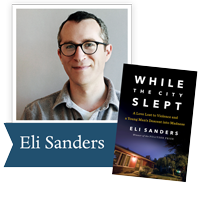A Pulitzer Prize–winning reporter’s gripping account of one young man’s path to murder—and a wake-up call for mental health care in America.

 table div table+table+table+table+table+table+table+table+table+table+table+table+table+table+table+table+table+table+table+table+table+table+table+table+table+table+table+table+table+table+table+table+table+table+table+table+table+table+table+table+table div table{width:100%;padding:0}table div table+table+table+table+table+table+table+table+table+table+table+table+table+table+table+table+table+table+table+table+table+table+table+table+table+table+table+table+table+table+table+table+table+table+table+table+table+table+table+table+table div table img{width:96.23%;padding:0;float:none}table div table+table+table+table+table+table+table+table+table+table+table+table+table+table+table+table+table+table+table+table+table+table+table+table+table+table+table+table+table+table+table+table+table+table+table+table+table+table+table+table+table div table td{width:100%;padding:0 1.88% 18px}/* styles */## Our 12th annual gala event is sold out, and will take place November 5, 2018.

Please click to see our upcoming 2017 public events.